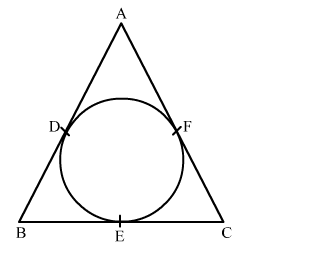# In the given figure, if AB = AC, prove that BE = CE.`
Question:

In the given figure, if AB = AC, prove that BE = CE.Solution:

Given, $A B=A C$

We know that the tangents from an external point are equal.

$\therefore A D=A F, B D=B E$ and $C F=C E \ldots \ldots . .(i)$

Now, $A B=A C$

$\Rightarrow A D+D B=A F+F C$

$\Rightarrow A F+D B=A F+F C \quad[$ from $(\mathrm{i})]$

$\Rightarrow D B=F C$

$\Rightarrow B E=C E \quad[$ from (i) $]$

Hence proved.